# Electronics and Communication Engineering - Exam Questions Papers

6.

The maximum percent overshoot with maximum value of first overshoot as 3.5 V and steady state value as 2.575 V is

 A. 35.92% B. 26.43% C. 57.61% D. 15.24%

Explanation:

From the transient response characteristics, Maximum percentage overshoot (MPO) is given as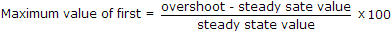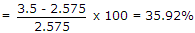MPO decides the relative stability of the system.

7.

Find Vo(t) for the following circuit.
Data: L = 1 H, C = 1 F, R1 = R2 = 1 Ω and zero initial conditions.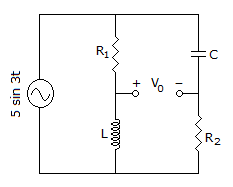A. 0 V B. 5 V C. 3 V D. 2 V

Explanation:

E(s) = L[e(t)] = L[5 sin 3t]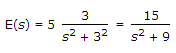Let V1(s) = Voltage across L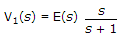and

V2(s) = Voltage across R2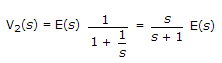V(s) = V1(s) - V2(s)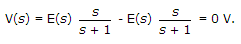8.

An XOR gate with 8 variable is as follows:
A ⊕ B ⊕ C ⊕ D ⊕ E ⊕ F ⊕ G ⊕ H
The number of minterms in the Boolean expression is

 A. 255 B. 127 C. 128 D. 256

Explanation: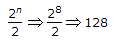.

9.

The figure shown below is designed to act as a constant current source across the load resistor. The β of transistor is 50. What is the value of load current?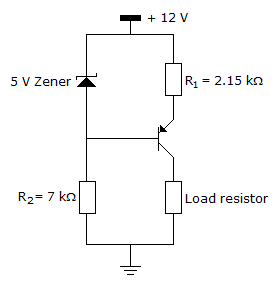A. 1.96 mA B. 2 mA C. 2.04 mA D. None

Explanation:

Voltage at base = 12 - 5 = 7 v

Voltage at emitter = 7 + 0.7 = 7.7 v

Current through R1 = 2 mA

Thus current through load =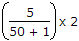= 1.96 mA.

10.

For a given magnetic material operating in linear mode B = 0.06 T. Assume permeability = 51, then magnetization is:

 A. 44836 A/m B. 46834 A/m C. 946 A/m D. 936 A/m

Explanation: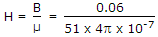μ = λmH,

λm = (μ - 1) = 51 - 1 = 50

μ = 50 x H46834.

#### Current Affairs 2022

Interview Questions and Answers